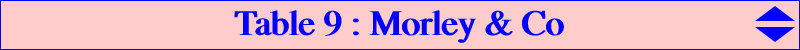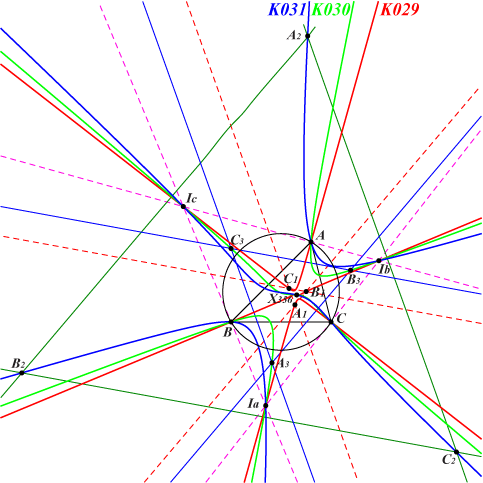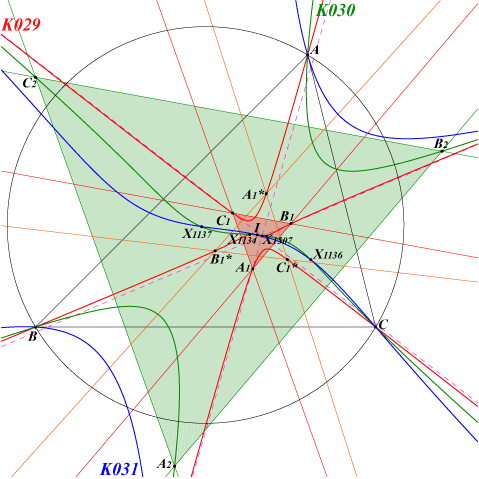Here is a comparative description of Morley triangles and cubics. This page was made with contributions by Edward Brisse. Denote by T1, T2, T3 the three Morley triangles and by T1*, T2*, T3* the three corresponding Morley adjunct triangles (see TCCT, pp. 165-167, §§ 6.25, 6.26, 6.27, 6.30). IaIbIc is the excentral triangle. Perspective triangles and perspectors :T1 T2 T3 T1* T2* T3* ABC is perspective at X(357) X(1134) X(1136) X(358) X(1135) X(1137)perspector T1 T1* X(356), centroid of T1 and pivot of K029 T2 T2* X(356)+ = X(3277), centroid of T2 and pivot of K030 T3 T3* X(356)- = X(3276), centroid of T3 and pivot of K031T1 T2 T3 T1* T2* T3* IaIbIc is perspective at X(1507) X(1507)+ X(1507)- X(1508) X(1508)+ X(1508)-Each point P related to T1 has a "plus" version P+ and a "minus" version P- obtained when the trisectors of A, B, C are replaced by those of A+2pi, B+2pi, C+2pi and A-2pi, B-2pi, C-2pi respectively. For example, X(1134) = X(357)+ and X(1136) = X(357)-. Points on the cubics :The Morley cubic contains A, B, C, X(1), Ia, Ib, Ic and : K029 K030 K031 the vertices of T1 and T1* T2 and T2* T3 and T3* its pivot, the center of Ti X(356) X(3277) = X(356)+ X(3276) = X(356)- the isogonal conjugate of its pivot X(3605) = X(356)* X(3607) = X(356)+* X(3606) = X(356)-* the perspector of ABC and Ti X(357) X(1134) X(1136) the perspector of ABC and Ti* X(358) X(1135) X(1137) the perspector of IaIbIc and Ti X(1507) X(1507)+ X(1507)- its isogonal conjugate X(1507)* X(1507)+* X(1507)-* the perspector of IaIbIc and Ti* X(1508) X(1508)+ X(1508)- its isogonal conjugate X(1508)* X(1508)+* X(1508)-* other centers X(1134), X(1135) X(1136), X(1137) X(357), X(358) other points cevians of X(356) cevians of X(356)+ cevians of X(356)-Related trilinear coordinates : The following table gathers together some points with first trilinear coordinate containing A/3 angles.t cos(A/3 + t) sin(A/3 + t) sec(A/3 + t) csc(A/3 + t) on the line X(16)-X(358) on the circum-conic through X(14), X(357) - π/3 X(1135) X(3274) X(1134) X(3602) - π/6 X(3273) X(1137) X(3604) X(1136) 0 X(358) X(3275) X(357) X(3603) π/6 X(3274) X(1135) X(3602) X(1134) π/3 X(1137) X(3273) X(1136) X(3604)Remark : the line X(16)-X(358) also contains all the points with first trilinear coordinate • cos A + 2 cos B/3 cos C/3 + t cos (A/3 + u), • cos A + 2 sin B/3 sin C/3 + t cos (A/3 + u), where t and u are any real numbers.Other related curves : See the pages K587, Q002, Q003, Q042, Q043.